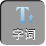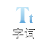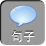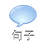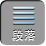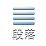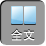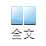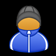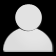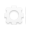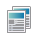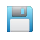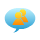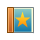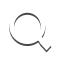-AA+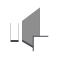“比例线段”的“学”和“用”

1）式子 ab= cd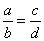，叫做比例式， abcd叫做比例项， ad叫做比例外项， bc叫做比例内项， d叫做 abc的第四比例项；

2）如果两个内项相等，即 ab= bcb叫做 ac的比例中项， c叫做 ab的第三比例项.

1）比例的基本性质：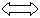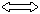ad= bc，特别地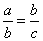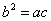.

2）等比性质：

1．下列四条线段中，不能成比例的是（）

Aa= 3b= 6c= 2d= 4

Ba= 1b=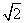c=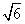d=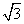Ca= 4b= 6c= 5d= 10

Da= 2b=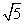c=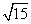d= 22．已知 xyz= 345，求（ 1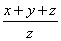；（ 2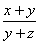；（ 3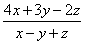的值

2=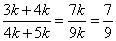3=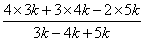=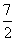.

3．如果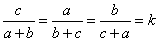，那么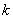=

2）当 a+ b+ c= 0时，则 a= b=－ｃ，ｂ＋ｃ＝－ a，ｃ＋ａ＝－ｂ，

1、已知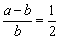，则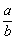=_____

2、已知 abc= 123，求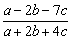得值

3．已知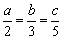，求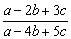的值

1.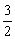2．因为 ab= 12，所以 b= 2 a，因为 ac= 13c= 3 a

3：设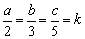，则 a= 2 kb= 3 kc= 5 k